# 1.2. Running the debugger

## 1. Prepare the project

Using the project from previous tutorial (blink), edit main.c to this little blinking demo:

/*
* main.c
*/

#define DELAY_ON	20000
#define DELAY_OFF	100000

static void 	reset_handler 		(void);
static void 	default_handler		(void);
int 			main 			(void);

/* Minimal vector table */
__attribute__ ((section(".isr_vector")))
void (* const table_interrupt_vector[])(void) =
{
(void *)0x20000800, 	// 0 - stack
reset_handler, 	 	// 1 - reset handler
default_handler, 	// 2 - NMI handler
default_handler, 	// 3 - Hardfault handler
};

/* Main program */
int main(void)
{
int i = 0, j = 0;
unsigned char state = 0;

*(int *)0x40021014 |=  (0x01 <<17U);
*(int *)0x48000000 &= ~(0xC00);
*(int *)0x48000000 |=  (0x01 <<10U);

while(1)
{
switch (state)
{
case 0:
{
*(int *)0x48000014 &= ~0x00000020U;
i++;
if (i>DELAY_OFF)
{
i = 0;
j++;
state = 1;
}
break;
}
case 1:
{
*(int *)0x48000014 |= 0x00000020U;
i++;
if (i>DELAY_ON)
{
i = 0;
state = 0;
}
break;
}
}
}
}

/* Reset handler */
void reset_handler (void)
{
main();
}

/* Default handler */
void default_handler(void)
{
for(;;);
}


Save alland build(or rebuild) the project. There must be no error or warning:Note that debugging is only possible when the project builds without error. If the project does not compile due to syntax errors for instance, then you must fix these errors before being able to start a debug session. To do that, all the help you have are the messages that the compiler outputs into the console. Therefore, debugger is only involved for tracking errors (bug) into “working” programs…

Take a look at the Build Analyzer window. It shows the amount of memory used by the current project. Note that only statically allocated variables are taken into account in the memory usage, therefore local variables such as i, j, or state are nowhere in this report. You can explore the Memory Details tab to verify this.## 2. Debug session

Make sure that the Nucleo board is connected to the computer and that you have a valid debug configuration (). Then start the debugger.

Give a try hitting the resume button. You should see the green LED flashing.

Suspend code execution, and then reset the debugger to the main() entry point:The debugger offers several interesting views of MCU internal state. These views can be opened using the Window →Show View menu:### 2.1. Step through disassembly code

Open theDisassembly view. It should open on the right side of the main editor, pointing the next machine instruction to be executed.Execute the first line of the C code by pressing the Step-Over buttononly once and observe the code pointer (arrow) in both C and disassembly window. The first line of the C program corresponds to 4 assembly code lines, therefore it required 4 machine cycles to execute (actually, 2 cycles for each variable (i, j) initialization).C source Assembly code

Next 3 assembly lines are supposed to store the value 0 into the memory location corresponding to the variable state. Let us decompose this simple process. To do that, first open the Register view.

Next toggle the Instruction Stepping Mode by clicking thebutton in the main toolbar.

The first assembly line performs adds r3, r7, #7. According to programming manual, this operation takes the content of r7, add the value 7 to this content, and store the result in r3. Open theRegisters view and step overthis line. Change the number format of the r3 value into Hex (right-click →Number Format)

Is it doing as expected?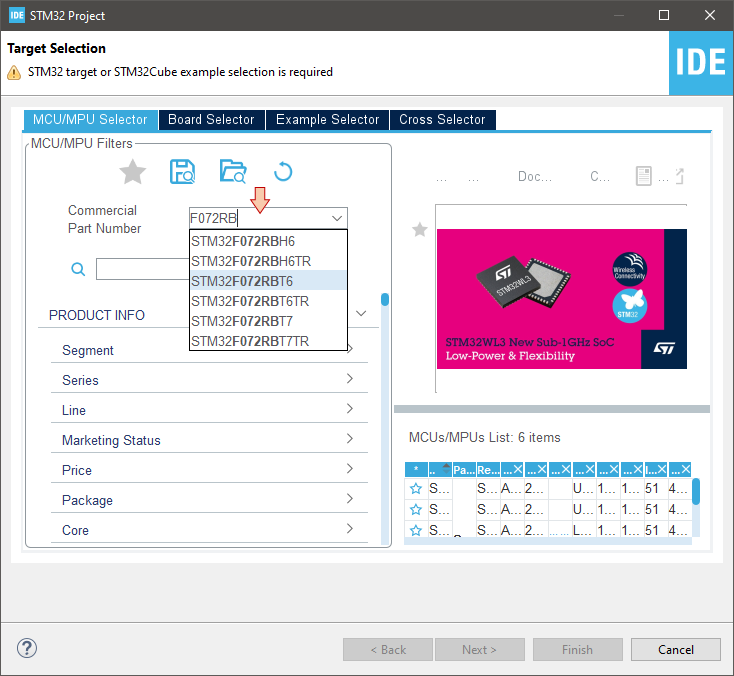r3 now holds the value 0x200007e7. This value looks like an adress in the user memory. It might be the adress of the state variable. Let us check.

Open theExpressions view. ClickAdd new expression and type ‘&state’. The tab now should look like this: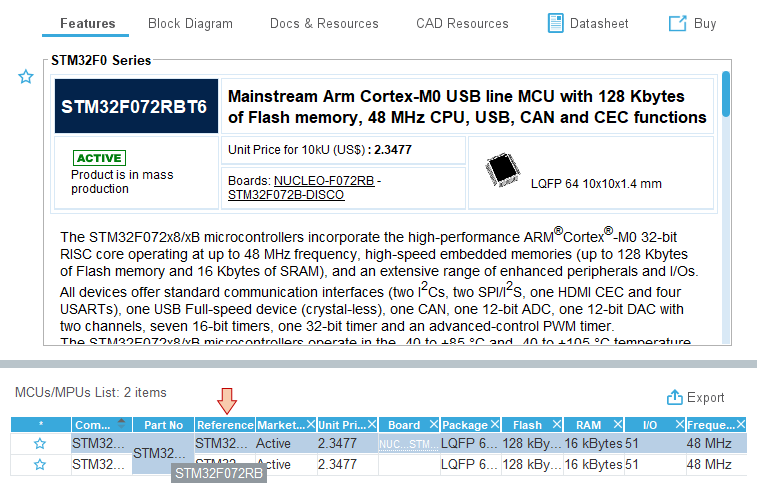By unfolding &state, you can also see *&state, which is nothing else than the value held by the state variable. The value of &state is the address in memory of the variable state. As expected, it is the same as the content of register r3.

Next assembly line is movs r2, #0. This should reset to 0 the content of r2. Step into the line and observe the Registry view to verify the effect of this line on r2.Next assembly line is strb r2, [r3, #0]. This instruction stores the content of r2 (i.e. 0) into the memory at address pointed by r3 with an offset 0 (therefore, at the address stored in r3). Note that if state variable is already 0, the line will have no effect.

Then, step-overone assembly line and make sure state is 0: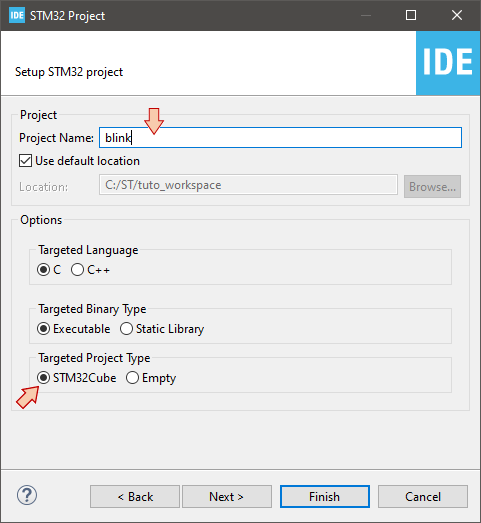From now on, let us stop stepping into disassembly code. Pressagain to deactivate the instruction stepping mode. At this moment, your debugger is stopped on the third line of the C program, just before just before some quite obscure initializations.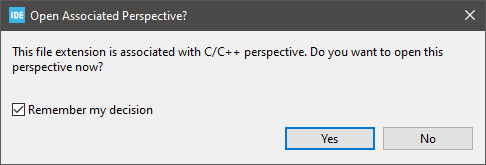Time for having a look into peripheral registers!

### 2.2. Monitoring peripheral registers

Open theSFRs view. A new tab will appear with a (long) list of all the STM32F072 peripherals.

Unfold the RCC peripheral: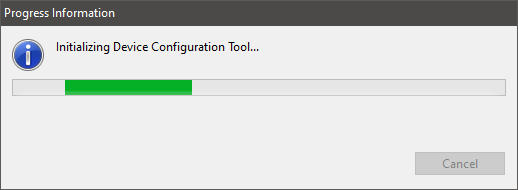Before stepping over the next code line, let us think about the meaning of it.

*(int *)0x40021014 |= (0x01 <<17U);

(int *)0x40021014   → (int*) is a pointer, therefore it is an address in memory
*(int *)0x40021014  → *(int*) is therefore the value that address holds
|=                  → is a bitwise logical OR between left and right operands
(0x01 <<17U)        → is the value ‘1’ left shifted (<<) by 17 bits

The int type represents 32-bit integers :
0x01 =

 0 0 0 0 0 0 0 0 0 0 0 0 0 0 0 0 0 0 0 0 0 0 0 0 0 0 0 0 0 0 0 1

(0x01 <<17U) =

 0 0 0 0 0 0 0 0 0 0 0 0 0 0 1 0 0 0 0 0 0 0 0 0 0 0 0 0 0 0 0 0

We don’t know the value stored @0x40021014, so let say X can be either 0 or 1
*(int *)0x40021014 =

 X X X X X X X X X X X X X X X X X X X X X X X X X X X X X X X X

Given the above, the operation:  *(int *)0x40021014 |= (0x01 <<17U) performs the following:

 X X X X X X X X X X X X X X X X X X X X X X X X X X X X X X X X

OR

 0 0 0 0 0 0 0 0 0 0 0 0 0 0 1 0 0 0 0 0 0 0 0 0 0 0 0 0 0 0 0 0

=

 X X X X X X X X X X X X X X 1 X X X X X X X X X X X X X X X X X

The result being stored at address 0x40021014

Put in simple words, the operation above does:
set bit number 17 of the register at address 0x40021014 to ‘1’ , and leave other bits as they are

The value (0x01 <<17U) is called a positive mask in the logical bitwise operation.

In theSFRs view, unfold the RCC AHBENR register. Then step-overonce and watch the IOPAEN bit. It should toggle from 0 to 1. By selecting that particular bit in the SFRs view, you get a short desciption of what it does.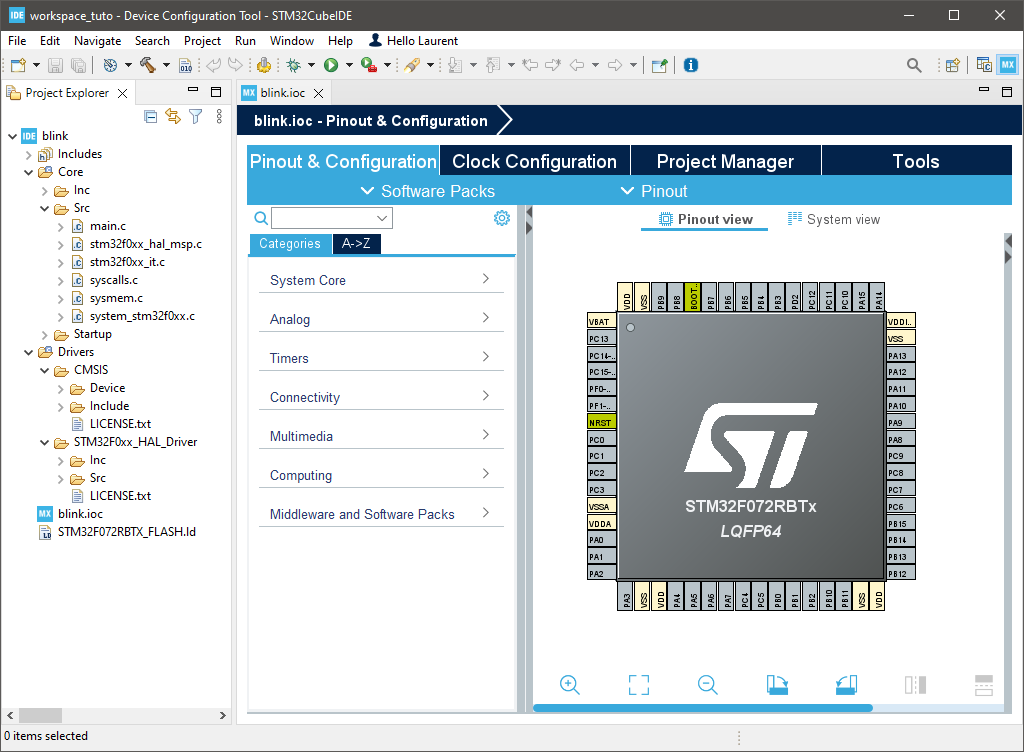You’ve just turned ON the clock of the General-Purpose Input Output (GPIO) pins associated to the port A (GPIOA). This is done in the Reset and Clock Control (RCC) peripheral.

You can find further information regarding this register in the Reference Manual:Next two lines are:


*(int *)0x48000000 &= ~(0xC00);
*(int *)0x48000000 |= (0x01 <<10U);

0xC00 =

 0 0 0 0 0 0 0 0 0 0 0 0 0 0 0 0 0 0 0 0 1 1 0 0 0 0 0 0 0 0 0 0

~(0xC00) =

 1 1 1 1 1 1 1 1 1 1 1 1 1 1 1 1 1 1 1 1 0 0 1 1 1 1 1 1 1 1 1 1

AND

*(int *)0x48000000 =

 X X X X X X X X X X X X X X X X X X X X X X X X X X X X X X X X

=
*(int *)0x48000000 =

 X X X X X X X X X X X X X X X X X X X X 0 0 X X X X X X X X X X

OR
(0x01<<10U) =

 0 0 0 0 0 0 0 0 0 0 0 0 0 0 0 0 0 0 0 0 0 1 0 0 0 0 0 0 0 0 0 0

=
*(int *)0x48000000 =

 X X X X X X X X X X X X X X X X X X X X 0 1 X X X X X X X X X X

In simple words, the operation is:
“set bits [11 10] of resister at address 0x48000000  to value ‘01’, leaving other bits as they are.”

Because leaving other bits unchanged requires a OR operation, we need first to set bits under interest to 0. That’s the purpose of the AND operation using ~(0xC00) as a negative mask.

Step-over the two lines and watch GPIOA registers: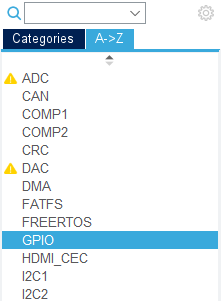Register at address 0x48000000 is called MODER. This is the register that defines the direction (input/output) of each MCU pins associated with GPIOs. What we’ve just done, is to set PA5 pin as an output.

Note that if the GPIO clock is not started before (as we did), MODER cannot be written. Again, you can refer to Reference Manual to get detailed information regarding the MODER register:### 2.3. Monitoring Memory

If everything goes well, the debugger is now stopped at the beginning of the infinite loop:Pressseveral times to verify that the switch statement behaves as expected (until i=3 for instance). Doing that, observe the values of state, i, j into theVariables tab.Open theMemory view. Adda monitor &i. The memory viewer then shifts to display the location of i variable first (here at address 0x200007ec).Step overthe code another couple of times to see if the value of i correctly changes in both the Variables and Memory views.

Suppose now that we want to check that the switch statement works well when i variable reaches 100000. Obviously, it would take too much time (and pain) to manually step through the code until then.

There are at least 3 ways to achieve this much faster:

• Change the value of i to something close to 100000 (eg 99999), in the Variables view
• Position editing cursor on line 42 (first instruction when i>100000) and then use Run→Run To Linemenu command
• Set a breakpoint at line 42, and then press the run buttonLet-us try the second method. Position editing cursor on line 42 and then use Run→Run To Linemenu command. Watch the value of i in theVariables view. Should be 100001 now.

Step-overthe code another couple of times, looking at i, j and state variables and make sure things are behaving as expected (now looping through the state 1).

Press the runbutton and verify that the LED is blinking in real time, as a normal code execution.

### 2.4. Using Breakpoints

Suspend the debuggerThe code line pointer is somewhere in the main loop, depending on when you pressed the pause button.  Let us assume that we want to stop the code execution each time there is a change in the LED state. We can do that with breakpoints.

Double-click in the vertical blue lane (on the left of the main editor frame) at line 44 and line 55. You have set two breakpoints, right before LED state changes:Now the LED state should toggle every time you press.

Note that breakpoints are a limited hardware feature of the MCU. STM32F072 offers up to 4 breakpoints. You cannot set as many breakpoints you want.

Info : stm32f0x.cpu: hardware has 4 breakpoints, 2 watchpoints

Terminate the debug session.

## 3. Summary

In this tutorial you have learned the use of the debugger for different purposes. Debugging is an essential process that you should get familiar with.
No new code (even apparently working) should not be released without a deep check (line by line) under debugger. You’ll be surprised…